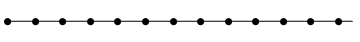# Alexandroff line

If this isn’t one of Ben Elliott and Hunter Spink’s bizarre mathematical objects of the week, it certainly deserves to be. This is a strange object which locally behaves like the real line, but is much longer. Instead of considering the entire real line, we’ll focus on the positive half-line:As shown above, it’s obtained by gluing together a countable set of intervals. In the diagram above, they are indexed by the ordinal ω. If we do the same thing for the ordinal ω², then we obtain the same ordinary real half-line:The fusible numbers give a way of gluing together ε_0 intervals to obtain the real half-line. We can find similar constructions for all countable ordinals. Can we find an uncountable well-ordered subset X of the real line? The answer is no. For every x in X, there is a unique successor, S(x). Between x and S(x), there exists a rational number f(x). Clearly, the function f must be injective, so X is at most countably infinite.

So, what happens if we glue together ω_1 intervals, where ω_1 is the first uncountable ordinal? This must be longer than the real line, but for every point α, the interval [0,α) is homeomorphic to the real half-line [0,∞), which is in turn homeomorphic to the semi-open interval [0,1). Hence, we have something that locally resembles the real half-line, known as the Alexandroff ray. Gluing together two of these, back-to-back, gives the Alexandroff line, which locally resembles the real line despite being infinitely longer in both directions.

And guess what? In the Cartesian product (Alexandroff line)^2, we can pack uncountably many figure-eights. Can we pack as many figure-eights into the Alexandroff plane as we can circles? The answer, of course, is ‘if and only if the continuum hypothesis is true’…

This entry was posted in Uncategorized. Bookmark the permalink.

### 0 Responses to Alexandroff line

1.lillysdjservice.com says:

Hello very nice blog!! Guy .. Excellent .. Superb ..
I’ll bookmark your blog and take the feeds also?
I am satisfied to find so many helpful info right
here in the post, we need develop more techniques on this regard, thank
you for sharing. . . . . .

2.delvalwebdev says:

Thanks for the good writeup. It actually was once a enjoyment account it.
Glance advanced to far brought agreeable from you! By the way, howw
could we communicate?